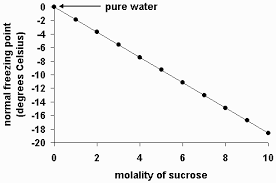## How to Calculate and Solve for Van’t Hoff’s Factor, Cryoscopic Constant, Molality and Freezing Point Depression | Nickzom CalculatorThe image above represents the freezing point depression.

To compute for the freezing point depression, three essential parameters are needed and these parameters are Van’t Hoff’s Factor (i), cryoscopic constant (Kf) and molality.

The formula for calculating freezing point depression:

δTf = iKf x Molality

Where;
δTf = Freezing point depression
i = Van’t Hoff’s Factor
Kf = cryoscopic constant
Molality

Let’s solve an example;
Find the freezing point depression when the van’t hoff’s factor is 12, cryoscopic constant is 21 with a molality of 16.

δTf = iKf x Molality
δTf = (12 x 21) x 16
δTf = 252 x 16
δTf = 4032

Therefore, the freezing point depression is 4032 °C m-1.